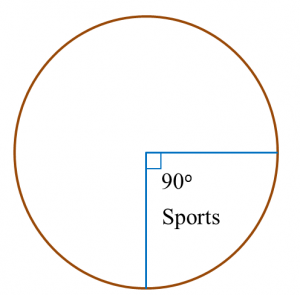# 12.2.3 Measures of Central Tendencies, PT3 Focus Practice

12.2.3 Measures of Central Tendencies, PT3 Focus Practice

Question 6:
Diagram below is a pie chart which shows the proportion of money allocated to five clubs.The total amount of money allocated to Swimming Club and Squash Club is RM1800.
Calculate the total amount of money allocated to the five clubs.

Solution
:
Sum of the angles of the sectors for Swimming Club and Squash Club
= 360o – 65o – 85o – 90o
= 120o

Total amount of money allocated to the five clubs
$\begin{array}{l}=\frac{{360}^{o}}{{120}^{o}}×RM1800\\ =RM5400\end{array}$

Question 7:
Table below shows the number of students who watch four different TV channels in a week.

 TV Channels Number of Students News 8 Sports 15 Movies 25 Music 12
The information for sports channel is shown fully in the pie chart in the answer space.
Complete the pie chart to represent all the information in the Table.$\begin{array}{l}\text{News channel}\\ =\frac{8}{60}×{360}^{o}={48}^{o}\\ \\ \text{Movies channel}\\ =\frac{25}{60}×{360}^{o}={150}^{o}\\ \\ \text{Music channel}\\ =\frac{12}{60}×{360}^{o}={72}^{o}\end{array}$